FreeBuf.COM网络安全行业门户，每日发布专业的安全资讯、技术剖析。FreeBuf+小程序

Java代码审计基础之反射
2020-07-10 02:08:40

## 反射类的首要操作 - 获取类

（1）直接根据类名获取类

`Class a = Cat.class;`

（2）通过Class.forName 获取类，需要打全指定类的路径

`Class a = Class.forName("org.xiaopan.test.Cat");`

Class.forName 需要一个异常处理。不然编辑器无法通过。
（3）通过实例化的方式获取类
`Class a = (new Cat()).getClass();`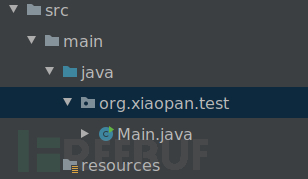## 反射构造方法

```class Cat{
//构造方法，形参为空
public Cat(){
System.out.println("Cat->nullCat");
}

//构造方法，形参为 一个String类型
public Cat(String a){
System.out.println("Cat->aCat,a=" + a);
}

//私有构造方法，形参为 一个String类型 和 一个Integer类型
private Cat(String a,Integer b){
System.out.println("Cat->abCat,a=" + a + "b=" + b.toString());
}

//构造方法，形参为 一个String数组类型 和 一个Map类型
public Cat(String[] aa,Map bb){
System.out.println("Cat->aabbCat");
System.out.println("aa=" + Arrays.toString(aa));
System.out.println("bb=" + bb);
}
}```

### 无参构造方法调用

```try{
//获取到类
Class a = Cat.class;
//通过反射获取到指定类下的构造方法
//要获取的构造方法为:
//public Cat()
//由于该构造方法无参数，所以我们传入一个 null 即可，也可以不传
Constructor constructor1 = a.getConstructor(null);
//实例化类
constructor1.newInstance(null);
}
catch (Exception e){
System.out.println(e);
}```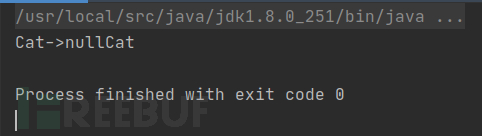getConstructor 需要加一个异常处理。

### 一个参数的构造方法调用

```try{
//获取到类
Class a = Cat.class;
//通过反射获取到指定类下的构造方法
//要获取的构造方法为:
//public Cat(String a)
//由于该构造方法有一 String类型 参数
//在进行 getConstructor 反射时，就需要指定传参类型为 String.class
Constructor constructor1 = a.getConstructor(String.class);
//在实例化时，进行传参
constructor1.newInstance("testvalue");
}
catch (Exception e){
System.out.println(e);
}```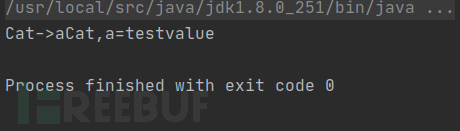### 多个参数的私有构造方法调用

```try{
//获取到类
Class a = Class.forName("org.xiaopan.test.Cat");
//通过反射获取到指定类下的构造方法
//要获取的 私有 构造方法为：
//private Cat(String a,Integer b)
//
//由于是 私有 方法，获取私有方法的函数为 getDeclaredConstructor
//由于有两个参数，所以需要在 getDeclaredConstructor 传入对应的参数类型
Constructor c = a.getDeclaredConstructor(String.class, Integer.class);
//设置强制反射
c.setAccessible(true);
//构造函数实例化，并传参
//这里有个注意点，看下文的注意
c.newInstance(new Object[]{"abcd",123456});
}
catch (Exception e){
System.out.println(e);
}```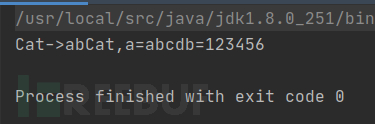jdk1.4中，数组每个元素对应一个参数
jdk1.5中，整个数组是一个参数，用一个对象包起来

```c.newInstance(new Object[]{"abcd",123456});
//即
new Object[]{"abcd",123456} 的格式，用一个对象包裹起来```

### 形参为数组和Map类型的构造方法调用

`String[] a = {"abc", "def"};`

Map：
java中的map，可以理解为“可自定义键值的数组”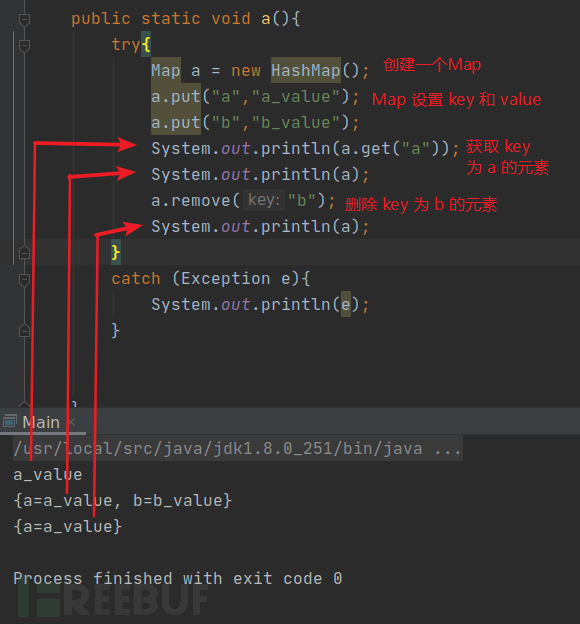```try{
//获取类
Class a = (new Cat()).getClass();
//调用的构造方法为：
//public Cat(String[] aa,Map bb)
//照常打 xxx.class 即可。java 万物皆对象
Constructor c = a.getConstructor(String[].class, Map.class);
//创建一个map
Map m = new HashMap();
m.put("a_key","a_value");
//实例化构造函数，注意要用 Object包裹的形式
//new String[]{} 是当场初始化字符串数组，当场赋值
c.newInstance(new Object[]{new String[]{"a","b","c"}, m});
}
catch (Exception e){
System.out.println(e);
}```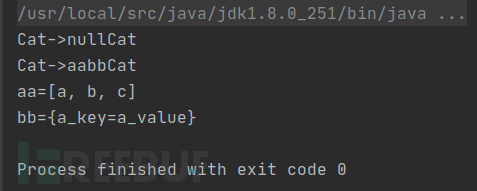## 反射方法

```class Cat{
public void a(){
System.out.println("a invoke");
}

public String[] b(String[] b){
return b;
}

public static void c(){
System.out.println("cccccc");
}
}```

### 反射无参数方法

```try{
//获取类
Class a = Class.forName("org.xiaopan.test.Cat");
//先实例化，后面调用方法的时候需要使用实例化好的类
//注意，实例化之后返回的类型就是对于的类，做好类型转换
Cat cat =(Cat) a.newInstance();
//调用的方法为：
//public void a()
//
//获取方法，需要指定要获取的方法名
Method m = a.getMethod("a", null);
//调用方法，调用方法时，用 上一步代码中获取到的方法进行 invoke 调用操作
//而invoke方法中，第一个参数是实例化好的类
//第二个参数就是需要传入的参数
m.invoke(cat,null);

}
catch (Exception e){
System.out.println(e);
}```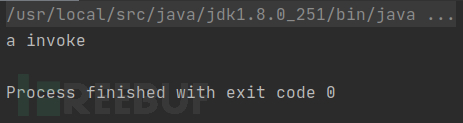### 反射有参数有返回值方法

```try{
//获取类
Class a = Class.forName("org.xiaopan.test.Cat");
//先实例化，后面调用方法的时候需要使用实例化好的类
//注意，实例化之后返回的类型就是对于的类，做好类型转换
Cat cat =(Cat) a.newInstance();
//调用的方法为：
//public String[] b(String[] b)
//
//获取方法，需要指定要获取的方法名
Method m = a.getMethod("b",String[].class);
//调用方法，调用方法时，用 上一步代码中获取到的方法进行 invoke 调用操作
//调用时，如果参数是字符串数组，或者两个以上的参数
//最好使用 new Object[]{} 的形式传入
//兼容性好
//由于有返回值，我们在调用的时候也需要进行接收
//接受类型就看调用的类返回的类型了
String[] strs = (String[]) m.invoke(cat, new Object[]{new String[]{"str1","str2","str3"}});

//打印数组：
//for each打印数组，先指定抽出来的元素类型
//然后以冒号 : 分隔，左边是抽出元素变量名，右边是原数组
for (String str:strs){
System.out.println(str);
}

}
catch (Exception e){
System.out.println(e);
}```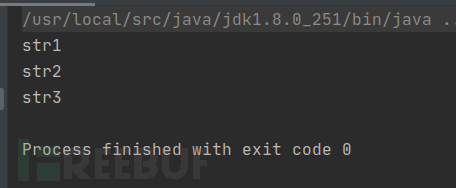### 反射静态方法

```try{
//获取类
Class a = Class.forName("org.xiaopan.test.Cat");
//调用的方法为：
//public static void c()
//
//获取方法
Method m = a.getMethod("c");
//由于是静态方法。直接调用，类对象中传入null即可
m.invoke(null);

}
catch (Exception e){
System.out.println(e);
}```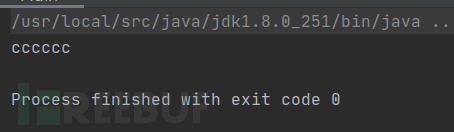## 反射属性

```class Cat{
public String name = "maomao";      //公共 String类型 属性
public static Boolean sex = true;   //公共 静态 String类型 属性
private Integer age = 10;           //私有 Integer类型 属性
}```

### 反射公共属性

```try{
//获取类
Class a = Class.forName("org.xiaopan.test.Cat");
//先实例化，后面获取属性的时候需要使用实例化好的类
Cat cat = (Cat) a.newInstance();
//属性：
//public String name = "maomao";
//
//获取属性
Field m = a.getField("name");
//获取属性值
//需要传入实例化类作为对象
String name = (String) m.get(cat);
System.out.println(name);

}
catch (Exception e){
System.out.println(e);
}```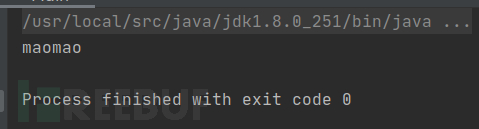### 反射公共静态属性

```try{
//获取类
Class a = Class.forName("org.xiaopan.test.Cat");
//属性：
//public static Boolean sex = true;
//
//获取属性
Field m = a.getField("sex");
//获取属性值,静态属性不需要实例化类，直接传入null作为类对象即可
Boolean b = (Boolean) m.get(null);
System.out.println(b);
//设置属性值
m.set(null,false);
b = (Boolean) m.get(null);
System.out.println(b);

}
catch (Exception e){
System.out.println(e);
}```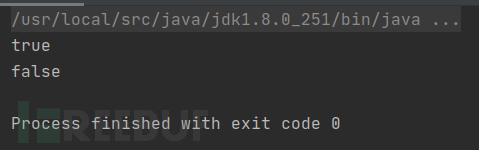### 反射私有属性

```try{
//获取类
Class a = Class.forName("org.xiaopan.test.Cat");
//先实例化，后面获取属性的时候需要使用实例化好的类
Cat cat = (Cat) a.newInstance();
//属性：
//private Integer age = 10;
//
//获取私有属性
Field m = a.getDeclaredField("age");
//设置强制反射
m.setAccessible(true);
//获取属性值
Integer age = (Integer) m.get(cat);
//注意输出的时候要将非String类型 toString 哦，规范一点
System.out.println(age.toString());
}
catch (Exception e){
System.out.println(e);
}```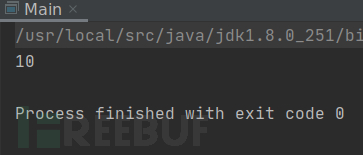## 引用包错误的报错：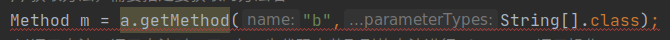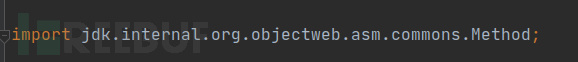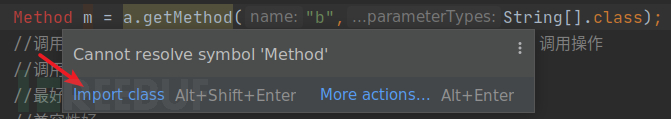## Runtime.getRuntime.exec 反射

### 了解 Runtime.getRuntime.exec

Runtime.getRuntime.exec 是Java中执行系统命令的方法

```byte[] a = new byte;

Process cmd = Runtime.getRuntime().exec("whoami");

InputStream input = cmd.getInputStream();
String res = new String(a);

System.out.println(res);```

### Process cmd = Runtime.getRuntime().exec("whoami")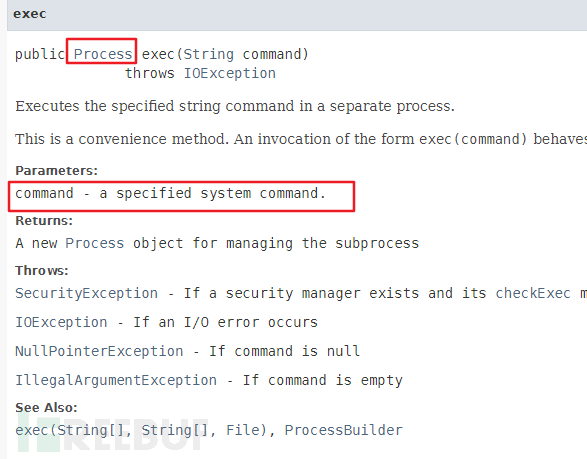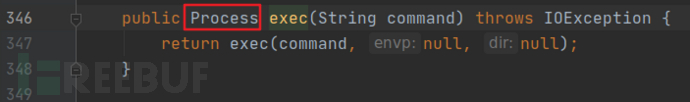Process类提供进程输入、输出等进程方法。粗浅的说就是一个进程类

### InputStream input = cmd.getInputStream()

InputStream    输入流，即数据流入，读入数据
OutputStream 输出流，即数据输出，写入数据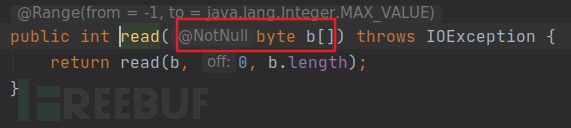String res = new String(a);

## 反射调用 Runtime.getRuntime().exec

### 第一种方式，通过强行反射私有构造方法，用 Runtime 实例化进行反射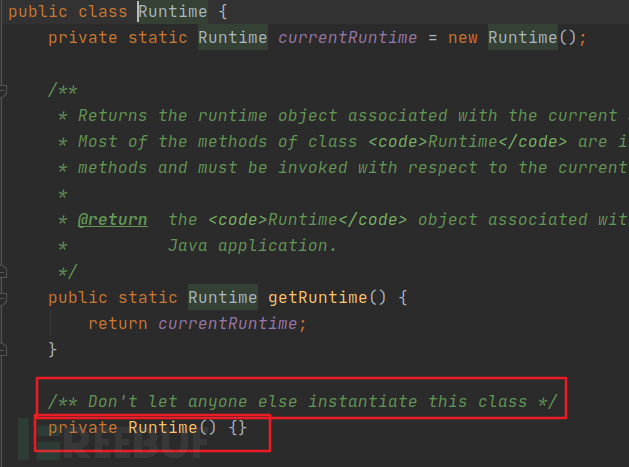```Class runtime = Class.forName("java.lang.Runtime");
runtime.newInstance();```

java.lang.IllegalAccessException: Class org.xiaopan.test.Main can not access a member of class java.lang.Runtime with modifiers "private"

```Class runtime = Class.forName("java.lang.Runtime");
Constructor c = runtime.getDeclaredConstructor();
c.setAccessible(true);
c.newInstance();```

```byte[] a = new byte;
try{
//获取Runtime类
Class runtime = Class.forName("java.lang.Runtime");
//获取Runtime类构造方法
Constructor c_runtime = runtime.getDeclaredConstructor();
//设置强制反射
c_runtime.setAccessible(true);
//Runtime类的构造方法 实例化
//由于 Runtime类构造方法返回类型为 Runtime类，所以需要使用 Runtime 类型变量进行接收
Runtime r = (Runtime) c_runtime.newInstance();
//获取 Runtime类的方法 exec
Method m = runtime.getMethod("exec", String.class);
//调用 exec 方法，传入对象为 Runtime类的构造方法实例化
//由于 exec 方法返回的是 Process 类型数据，所以需要使用 Process 类型变量进行接收
Process p = (Process) m.invoke(r,"whoami");
//读入数据流，读入到 byte[] 类型的变量中
System.out.println(new String(a));
}
catch (Exception e){
System.out.println(e);
}```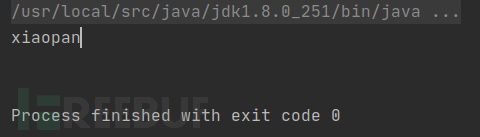### 第二种方式，不进行 Runtime实例化，直接通过getRuntime进行反射

`Runtime.getRuntime().exec("whoami");`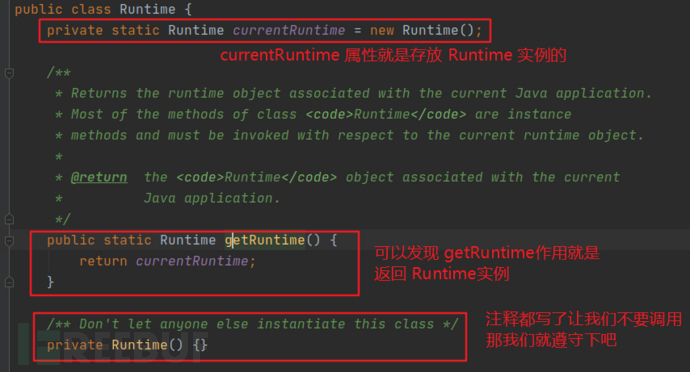```byte[] a = new byte;
try{
//获取 Runtime 类
Class runtime = Class.forName("java.lang.Runtime");
//获取getRuntime方法
Method m = runtime.getMethod("getRuntime");
//调用getRuntime方法，并用 Runtime类 类型进行接收，显式转换成 Runtime类
//调用的时候，在上文查看源码时，发现是不需要传入参数的
//不需传入参数，我们直接传一个 null 进去即可
Runtime r = (Runtime) m.invoke(null);
//由于上一个代码中调用 getRuntime 方法，返回了 Runtime类
//我们直接就可以调用底下的 exec 方法了
Process p = r.exec("ifconfig");
//数据输入流，读入数据
InputStream res = p.getInputStream();
System.out.println(new String(a));
}
catch (Exception e){
System.out.println(e);
}```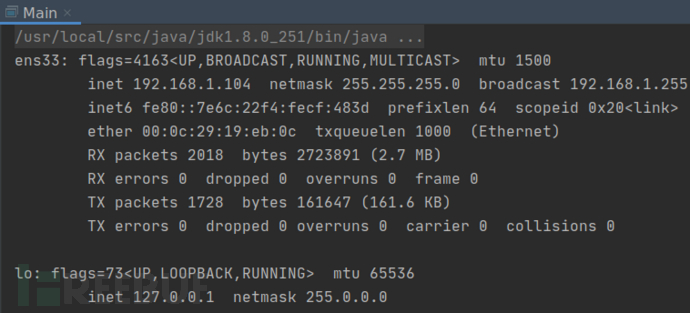# java # Java代码审计 # java反射

+ 收入我的专辑
+ 加入我的收藏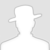• 0 文章数
• 0 评论数
• 0 关注者